American Journal of Physics and Applications
Volume 4, Issue 5, September 2016, Pages: 134-139

Thermal Performance of Electrocaloric Refrigeration using Thermal Switches of Fluid Motion and Changing Contact Conductance

Shigeki Hirasawa*, Tsuyoshi Kawanami, Katsuaki Shirai

Department of Mechanical Engineering, Kobe University, Kobe, Japan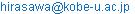(S. Hirasawa)

*Corresponding author

Shigeki Hirasawa, Tsuyoshi Kawanami, Katsuaki Shirai. Thermal Performance of Electrocaloric Refrigeration using Thermal Switches of Fluid Motion and Changing Contact Conductance.American Journal of Physics and Applications. Vol. 4, No. 5, 2016, pp. 134-139. doi: 10.11648/j.ajpa.20160405.12

Received: August 23, 2016; Accepted: September 7, 2016; Published: September 19, 2016

Abstract: Thermal performance of electrocaloric refrigeration system composed with a thin electrocaloric material and thermal switches was numerically calculated. Two types of thermal switches were studied: a thermal switch of fluid motion and a thermal switch by changing the contact thermal conductance. The following results were obtained. For the thermal switch of fluid motion with the frequency 10 Hz, the thicknesses of the electrocaloric material 200 μm and the water flow channel 100 μm, the average heat transfer efficiency was 11%. For the thermal switch by changing contact thermal conductance with the frequency 1000 Hz, the thicknesses of the electrocaloric material 20 μm and the heat storage material 20 μμ, the average heat transfer efficiency was 6% and the average heat flux transferred to the cold side of the system was 7 x 104 W/m2.

Keywords: Electrocaloric Refrigeration, Thermal Switch, Fluid, Contact, Thermal Resistance, Heat Transfer, Efficiency

1. Introduction

Recently new cooling technology using the electrocaloric effect in thin films of an electrocaloric material has attracted interest because of high energy efficiency. Figure 1 shows a basic model of the electrocaloric refrigeration system. A thin electrocaloric material was coupled with two thermal switches working at high temperature and working at low temperature. The electrocaloric material alternatively generated heat energy and cold energy by changing electric field. Here, cold energy means negative heat energy. The generated heat energy was transferred to the hot side of the system, and the cold energy was transferred to the cold side separately by the operation of thermal switches. The coupling of multi-layers of thin films of electrocaloric material with thermal switches can work as the efficient refrigerator. It is easy to enhance the cooling rate of the electrocaloric refrigerator by increasing the frequency of the change of the electric field.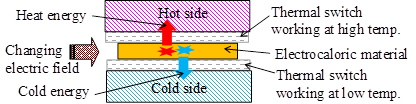Figure 1. Model of electrocaloric refrigeration system.

Radebaugh et al.  reported the feasibility of electrocaloric refrigeration for the 4 - 15 K temperature range. They studied two types of thermal switches of a multiple-leaf contact switch and a magneto thermal switch. Epstein and Malloy  reported a thin thermal-switching device using liquid crystals. Jia and Ju  reported experimental results of thermal performance of an electrocaloric refrigeration system using a mechanical thermal switch at a frequency of 0.1 Hz. Sato  reported the thermal switching behavior of a heat pipe. Guo et al.  designed an electrocaloric refrigeration system with a thermal switch of fluid motion. They reported that cooling power density was 3 × 104 W/m2 and a percent of Carnot COP (coefficient of performance) was 31% at a temperature span of 15 K and a frequency of 15 Hz. Ozbolt et al.  reviewed the technologies for the electrocaloric refrigeration. Hirasawa et al. [7 - 9] reported the thermal switching behavior of a flat heat pipe, changing the contact thermal conductance and fluid motion. They reported that the system using a fluid motion thermal switch increased the average heat transfer efficiency to 67 % at a frequency of 5 Hz for constant heat generation. They also reported that pulse heat generation was preferable to increase thermal performance . For practical use of electrocaloric refrigeration to cool electronic devices, more studies are still needed to understand the thermal performance at high frequency and the design guideline to increase performance of the electrocaloric refrigeration.

In this work, the thermal performance of electrocaloric refrigeration system with two types of thermal switches was numerically calculated for the pulse heat generation. They were a thermal switch of fluid motion and a thermal switch by changing the contact thermal conductance. Conditions to increase performance of the electrocaloric refrigeration were clarified.

2. Calculation Method for Thermal Switch of Fluid Motion

A model of the electrocaloric refrigeration system with a thermal switch of fluid motion was shown in Figure 2 . Both sides of a flat thin film of the electrocaloric material contact the hot water and cold water as they flow in thin-flow channels. The working diagram of the electrocaloric material and fluid motion was shown in Figure 3. The electrocaloric material generates heat energy and cold energy alternately by pulse heat generation with time interval te. The heat generating period of the pulse heat generation was assumed to be (te / 10). In this system, the fluid motion works as the thermal switch. When the electrocaloric material generates heat energy, water flows in the upper channel and air is present in the lower channel. The water in the upper channel transfers the heat energy to the hot side of the system. When the electrocaloric material generates cold energy, water flows in the lower channel and air is present in the upper channel. The water in the lower channel transfers the cold energy to the cold side of the system. Therefore, the heat energy and the cold energy transfer to the hot side and the cold side of the system separately.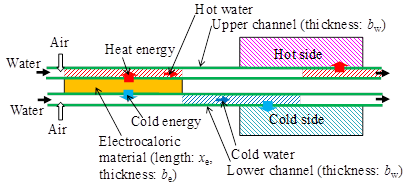Figure 2. Model of electrocaloric refrigeration system with thermal switch of fluid motion.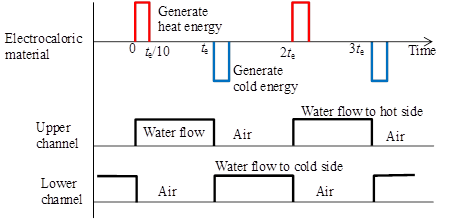Figure 3. Working diagram of electrocaloric material and fluid motion.

The high-frequency behavior of the cooling system is important to enhance the cooling rate of the refrigerator using the electrocaloric effect. However, the material has a thermal time-constant with respect to change temperature according to the size. The order of time-constant tc (s) for thermal conduction in a film is estimated by tc (ρ c / λ) b2 . Here, ρ is the density (5800 kg/m3 for BaTiO3 electrocaloric material  and 1000 kg/m3 for water ), c is the specific heat (430 J/kg K for electrocaloric material and 4200 J/(kg K) for water), λ is the thermal conductivity (2.6 W/m K for electrocaloric material and 0.62 W/(m K) for water), and b is the thickness of the film (m). The frequency of the changing electric field F (Hz) should be determined within the order of the thermal time-constant tc to keep good efficiency, and it is estimated by F 1 / (2 tc). The average heat flux qg (W/m2) of the generated heat energy in the electrocaloric material film was estimated by qg (Qg be F). Here, be is the thickness of the electrocaloric material film, Qg is the generated heat per unit volume for each change in the electric field, and F is the frequency of changing the electric field. The representative values of the heat change per unit volume for each change in the electric field were reported to be Qg = 6×107 J/m3 for a change in the electric field of 1.8×108 V/m .

Figure 4 shows the calculation results of the maximum thicknesses of the electrocaloric material film be, water flow channel bw within the limit of the thermal time-constant to change temperature, and the average heat flux qg of the generated heat energy in the electrocaloric material film.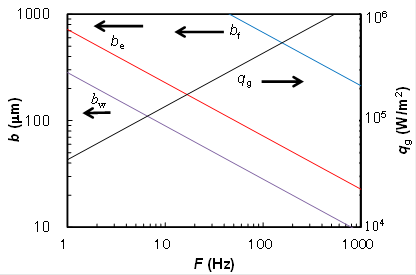Figure 4. Maximum thicknesses of electrocaloric material, water flow channel, heat storage material, and average heat flux of generated heat energy.

When the thermal switch was made by fluid motion in a thin-flow channel, the order of pressure drop ΔPfluid of the fluid caused by the inertia and viscosity was estimated by ΔPfluid {ρ (xe / tc)2 + μ xe2 / (tc bw2)} . Here, ρ is the density, μ is the viscosity, tc is the moving time, bw is the thickness of flow channel, and xe is the length of flow channel. Figure 5 shows the order of the pressure drop ΔPfluid for the water. In the calculation the thickness of flow channel bw was obtained from Figure 4, and the length of the flow channel was assumed to be xe = 0.1 m. In a practical condition the pressure drop ΔPfluid should be less than the order of 105 Pa. Then, the maximum frequency became on the order of F = 20 Hz from Figure 5. In this work, the case of the frequency F = 10 Hz and the time interval of pulse heat generation te = {1 / (2 F)} = 0.05 s were studied for a thermal switch of fluid motion. The maximum thicknesses of the electrocaloric material film and water flow channel became on the order of be = 200 μm and bw = 100 μm for F = 10 Hz from Figure 4. In this work, the case of be = 200 μm and bw = 100 μm were studied. The generated heat flux in the electrocaloric material film in generating period of (te / 10) is qe = {Qg be / (te / 10)} = 2.4 × 106 W/m2 for the generated heat per unit volume for each change in the electric field Qg = 6 × 107 J/m3. The average heat flux of the generated heat energy of the electrocaloric material film for the whole time period became on the order of qg = 1.2×105 W/m2. Also, the maximum temperature change of the electrocaloric material without any heat transfer is ΔT = {Qg / (ρ c)} = 24 K.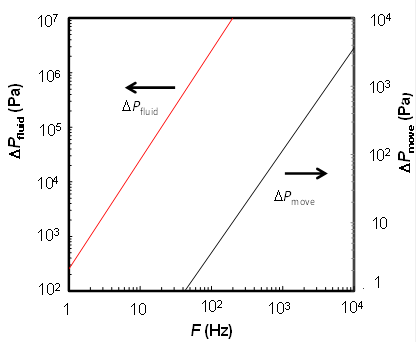Figure 5. Pressure drop of water flow and pressure to move heat storage material film.

The energy equations of the water flow and the electrocaloric material film were similar to our previous report .

In this work, the water flow velocity was assumed to be u = 8 m/s, which means that the flow time to pass the width of the electrocaloric material xe = 0.1 m is 0.013 s and it is less than the time interval of heat generation te. Inlet temperature of the hot water was assumed to be 5 K, inlet temperature of the cold water was assumed to be -5 K, and temperature difference between the hot water and cold water was about (Th - Tc) = 10 K for practical use of electrocaloric refrigeration. In this work temperatures were shown with the difference from the average temperature of the electrocaloric refrigeration system.

Heat transfer efficiency h was defined as the ratio between the average heat transfer rate to the cold side of system qc-ave and the generated cold energy in the electrocaloric material qg.

The equations were numerically calculated using the finite difference method . The number of calculation meshes was 10 to the flow direction, and 5 to the thickness direction (they were Th, Tek-h, Te, Tek-c, and Tc). The calculation time step was 1/10 of the heat generating time te.

3. Calculation Results of Thermal Performance for Thermal Switch of Fluid Motion

Figure 6 shows the calculation results of the temperature changes of the hot water Th at the exit side, the cold water Tc, and the electrocaloric material Te. The temperature of the electrocaloric material film Te increased when it generated heat energy, and it decreased when it generated cold energy. The temperature of hot water at the exit side Th was higher than the inlet temperature 5 K and the temperature of cold water at the exit side cold water Tc was lower than the inlet temperature -5 K. The average heat transfer efficiency was h = 11%, and the average heat flux transferred to the cold side of the system was qc-ave = 1.3 × 104 W/m2. The average heat transfer efficiency was less than 50% because some heat was consumed by the temperature change of the electrocaloric material.

If the thermal switch of fluid motion was the case of changing only the water flow velocity without air, the average heat transfer efficiency was less than zero. It is because there is the temperature difference of (Th - Tc) = 10 K between the hot water and the cold water. If the cold water stayed in the flow channel during the generation of heat energy in the electrocaloric material film, the cold water was heated to decrease the efficiency. Therefore the system of changing air and water flow was indispensable for the thermal switch of fluid motion in practice.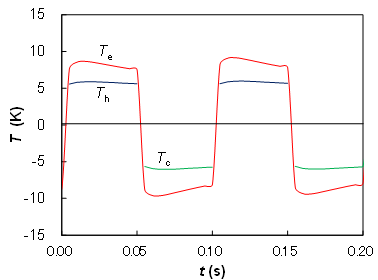Figure 6. Temperature change for thermal switch of fluid motion.

4. Calculation Method for Thermal Switch by Changing Contact Thermal Conductance

Figure 7 shows the calculation model for the electrocaloric refrigeration system with thermal switches by changing the contact thermal conductance. The electrocaloric material film and heat storage material films are sandwiched together. There are four thermal switches among the electrocaloric material film, heat storage material films, hot side, and cold side of the system. The operation of the thermal switches is to change the contact thermal conductance. When the distance between both walls of the thermal switch is less than 1 μm, the thermal conductance is high and heat flows through the thermal switch. When the distance between both walls is larger than 50 μm, the thermal conductance is low and the thermal switch is an insulator. Change of the distance between both walls can be achieved by movement of the heat storage material films driven by changing the pressure in the space to a vacuum or atmospheric pressure [1, 8]. The movement of the heat storage materials is small (in the order of 50 μm), and the working response is very high. Also thin thermal switches using liquid crystals, magneto thermal switches, and MEMS devices were studied [1,2].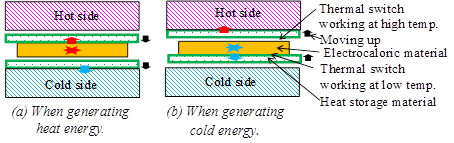Figure 7. Model of electrocaloric refrigeration system with thermal switch by changing contact thermal conductance.

The working diagram of heat generation in electrocaloric material and thermal conductance of thermal switches is similar to Figure 3. The electrocaloric material generates heat energy and cold energy alternately by pulse heat generation with time interval te and frequency F. After the generation of heat energy in the electrocaloric material, the thermal switch working at high temperature has high thermal conductance, and the heat energy transfers to the upper heat storage material. At that time, the thermal switch working at low temperature is the insulators. On the other hand, after the generation of cold energy in the electrocaloric material, the thermal switch working at low temperature has high thermal conductance, and the cold energy transfers to the lower heat storage material. At that time, the thermal switch working at high temperature is insulators. The heat energy in the upper heat storage material transfers to the hot side of the system during generation of cold energy in the electrocaloric material because of the operation of the thermal switch between the upper heat storage material and the hot side. The cold energy in the lower heat storage material transfers to the cold side of the system during generation of heat energy in the electrocaloric material. Therefore, the heat energy transfers to the hot side of the system, and the cold energy transfers to the cold side separately. In this work, the heat transfer phenomena of working fluids at the hot side and the cold side of the system were not calculated, and the temperatures Th, Tc of the hot side and the cold side of the system were assumed to be constant.

The heat storage material is an aluminum film (density ρ = 2700 kg/m3, specific heat c = 900 J/kg K, and thermal conductivity λ = 220 W/m K). The maximum thickness of heat storage material bf was calculated with the equations of time-constant and shown in Figure 4. The maximum thickness within the limit of the thermal time-constant to change the temperature of the heat storage material for the frequency F = 1000 Hz was in the order of bf = 200 μm and the maximum thickness of the electrocaloric material film was be = 20 μm.

When the operation of the thermal switch is driven by the movement of the heat storage material film caused by changing pressure, the required pressure ΔPmove to move distance ba in time tc is estimated with Eq. (1). It is the inertia force of the heat storage material film with thickness bf and densityρ .

ΔPmove ρ bf (ba / tc2)  (1)

The order of the pressure ΔPmove calculated with Eq. (1) is shown in Figure 5. In the calculation the thickness of heat storage material film bf was obtained from Figure 4, and the move distance was assumed to be ba = 50 μm. The pressure ΔPmove should be less than 105 Pa, which is the difference between vacuum and atmospheric pressure, and the maximum frequency was greater than F = 104 Hz from Figure 5. In this work, the following conditions were studied: the frequency F = 1000 Hz, the time interval to generate pulse heat generation was te = 0.0005 s, the thicknesses of electrocaloric material be = 20 μm, the aluminum heat storage material bf = 20 μm, the generated heat per unit volume for each change in the electric field Qg = 6 × 107 J/m3, the generated heat flux in the electrocaloric material in generating period was qe = 2.4 × 107 W/m2, the average generated heat flux in electrocaloric material was qg = 1.2 × 106 W/m2, the changing thermal conductance of the thermal switch was Ca = 5 × 104 W/m K and 500 W/m K (which corresponded to the distance between both walls of the thermal switch ba = 0.5 μm and 50 μm), and temperature difference between the hot side and the cold side of the system was (Th - Tc) = 10 K.

The energy equation of the electrocaloric material film is as follows.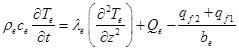(2)

Here, Te is the temperature of electrocaloric material film, Qe is the generated heat per unit volume due to the electrocaloric effect (W/m3), be is the thickness of the electrocaloric material, and qf2 and qf1 (W/m2) are the heat fluxes transferred to the upper and lower heat storage material, respectively.

The energy equation of the heat storage material film is as follows. The temperature distribution in the heat storage material was neglected because of large thermal conductivity.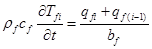(3)

Here, Tfi is the temperature of the i-th heat storage material, bf is the thickness, and qfi and qf(i-1) are the inlet heat fluxes transferred to the heat storage material.

The thermal conductance of thermal switch Ca (W/m2 K) was assumed to be only thermal conduction in the air between both walls of the thermal switch. The heat flux through thermal switch qfi (W/m2) from the electrocaloric material to the heat storage material is as follows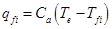(4)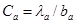(5)

Here, ba is the distance between both walls of the thermal switch (0.5 μm or 50 μm), and λa is the thermal conductivity of air (0.026 W/m K).

These equations were numerically calculated using the implicit finite difference method. The number of calculation meshes was 7 in the thickness direction z (they were Th, Tf2, Tek-h, Te, Tek-c, Tf1, and Tc).

5. Calculation Results of Thermal Performance for Thermal Switch by Changing Contact Thermal Conductance

Figure 8 shows the calculation results of the temperature changes of the electrocaloric material film Te, the heat storage material films Tf1, Tf2, the hot side Th, and the cold side Tc of the electrocaloric refrigeration system with thermal switch by changing contact thermal conductance. The temperature of electrocaloric material film Te was higher than the upper heat storage material film Tf2 and heat energy transferred to the upper heat storage material film when they generated heat energy. And the temperature of electrocaloric material film Te was lower than the lower heat storage material film Tf1 and cold energy transferred to the lower heat storage material films when they generated cold energy. Figure 9 shows the changes of the generated heat flux in each electrocaloric material qe (= ±2.4 × 107 W/m2), heat flux transferred to the hot side qh and the cold side qc of system. The cold heat transferred to the cold side of the system during generation of heat energy in the electrocaloric material because of the operation of the thermal switch between the lower heat storage material and the cold side. The average heat transfer efficiency was η = 6%, and the average heat flux transferred to the cold side of the system was qc-ave = 7 × 104 W/m2. The average heat transfer efficiency for the system with the thermal switch by changing contact thermal conductance was lower than that for the thermal switch of fluid motion, but the average heat flux transferred to the cold side of the system was higher because of the high frequency.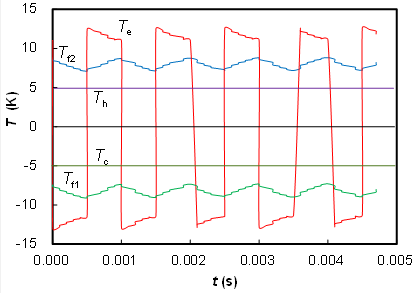Figure 8. Temperature change for thermal switch by changing contact thermal conductance.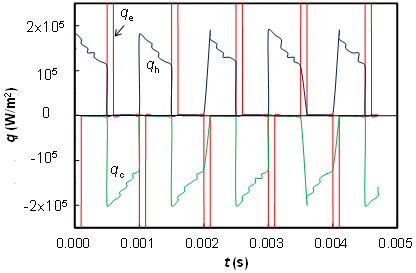Figure 9. Change of heat flux for thermal switch by changing contact thermal conductance.

6. Conclusions

The thermal performance of electrocaloric refrigeration with two types of thermal switches was numerically calculated: a thermal switch of fluid motion and a thermal switch by changing the contact thermal conductance. The following results were obtained.

(1)  The design guidelines of the electrocaloric refrigeration system were clarified. For the thermal switch of fluid motion, the maximum frequency was on the order of 20 Hz. The system of changing air and water flow was indispensable for the thermal switch of fluid motion in practice. For the thermal switch by changing contact thermal conductance, the maximum frequency was greater than 104 Hz.

(2)  For the thermal switch of fluid motion with the frequency 10 Hz, the thicknesses of the electrocaloric material 200 μm, the flow channel 100 μm, generated heat per unit volume for each change in the electric field 4 × 107 J/m3, and the temperature difference between the hot water and cold water 10 K, the average heat transfer efficiency was 11% and the average heat flux transferred to the cold side of the system was 1.3 × 104 W/m2.

(3)  For the thermal switch by changing contact thermal conductance with the frequency 1000 Hz, the thicknesses of the electrocaloric material 20 μm and the heat storage material 20 μm, the changing thermal conductance of the thermal switch is 5 × 104 W/m K and 500 W/m K, and temperature difference between the hot side and the cold side 10 K, the average heat transfer efficiency was 6% and the average heat flux transferred to the cold side of the system was 7 × 104 W/m2.

Acknowledgments

Authors thank to Mr. Makoto Endo, Mr. Naoki Fujimoto, Mr. Yusuke Satake, and Mr. Katsuhisa Hayashi of Kobe University for helping analysis and experiment.

Nomenclature

b   thickness of film, μ

Ca   thermal conductance, W/m K

c   specific heat, J/kg K

F   frequency, Hz

Qg   generated heat for each change in electric field, J/m3

q  heat fluxes, W/m2

qg  average heat flux of generated heat energy, W/m2

T  temperature, K

t   time, s

tc  time-constant or moving time, s

te  time interval of heat generation, s

xe   length of flow channel, m

z   coordinate to thickness, m

Δpressure, Pa

η   heat transfer efficiency

λ   thermal conductivity, W/m K

ρ   density, kg/m3

μ   viscosity, Pa s

Subscripts

a  air

c  cold side

e  electrocaloric material film

ek  surface of electrocaloric material film

heat storage material film

h  hot side

References

1. R. Radebaugh, W. N. Lawless, J. D. Siegwarth, A. J. Morrow, " Feasibility of Electrocaloric Refrigeration for the 4-15 K Temperature Range, Cryogenics, " vol. 19, 1979, pp. 187-208.
2. R. I. Epstein, K. J. Malloy, " Electrocaloric Devices Based on Thin-Film Heat Switches, " Journal of Applied Physics, vol. 106, 064509, 2009.
3. Y. Jia, Y. S. Ju, " A Solid-State Refrigerator B ased on the Electrocaloric Effect, " Applied Physics Letters, vol. 100, 242901, 2012.
4. W. Sato, " A Study of a New Cooling Device Based on the Electrocaloric Effect, " Proc. of the ASME 2013 International Mechanical Engineering Congress, San Diego, IMECE2013-62258, 2013.
5. D. Guo, J. B. Gao, Y. J. Yu, S. Santhanam, A. Slippey, G. K. Fedder, A. J. H. McGaughey, S.C. Yao, " Design and Modeling of a Fluid-Based Micro-Scale Electrocaloric Refrigeration System, " International Journal of Heat and Mass Transfer, vol. 72, 2014, pp. 559-564.
6. M. Ozbolt, A. Kitanovski, J. Tusek, A. Poredos, " Electrocaloric Refrigeration: Thermodynamics, State of the Art and Future Perspectives, " International Journal of Refrigeration, vol. 40, 2014, pp. 174-188.
7. S. Hirasawa, T. Nakamu, T. Kawanami, K. Shirai, " Study on Unsteady Thermal-Switching Function of Flat Heat Pipe, " Proc. of the ASME 2015 International Mechanical Engineering Congress & Exposition, 2015, Houston, IMECE2015-50158, 2015.
8. S. Hirasawa, S. Fujimoto, T. Nakamu, T. Kawanami, K. Shirai, " Experiment on Heat Transfer Control by Changing Contact Thermal Resistance, " Proc. of the JSME 2014 Mechanical Engineering Congress, Tokyo, G0610101, 2014 (in Japanese).
9. S. Hirasawa, T. Kawanami, K. Shirai, " Efficient Cooling System using Electrocaloric Effect, " Journal of Electronics Cooling and Thermal Control, vol. 6, 2016, pp. 78-87.
10. Y. He, "Heat Capacity, Thermal Conductivity, and Thermal Expansion of Barium Titanate-Based Ceramics," Thermochimica Acta, vol. 419, 2004, pp. 135–141.
11. J. P. Holman, Heat Transfer, 9th Ed., McGraw-Hill International Book Co., Boston, 2002.
12. G. Zhang, Q. Li, H. Gu, S. Jiang, K. Han, M. R. Gadinski, M. A. Haque, Q. Zhang, Q. Wang, " Ferroelectric Polymer Nanocomposites for Room-Temperature Electrocaloric Refrigeration, " Advance Material, vol. 27, 2015, pp. 1450-1454.

 Contents 1. 2. 3. 4. 5. 6.
Article ToolsAbstractPDF(303K)